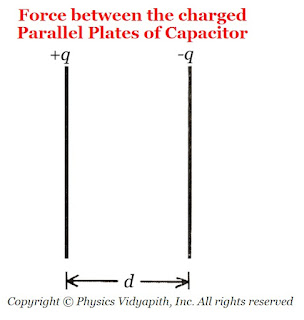### Force between the plates of a Charged Parallel Plate Capacitor

Derivation and Description→

Consider, A parallel plate capacitor with a charge $+q$ on one of its plates and $-q$ on the other plate. Let initially the plates of the capacitor are almost, but not quite touching. Due to opposite polarity, there is an attractive force $F$ between the plates. Now If these plates are gradually pulling and apart to a distance $d$, in such a way that $d$ is still small compared to the linear dimension of the plates, then the approximation of a uniform field between the plates is maintained and thus the force remains constant.The force between the charged parallel plates of the capacitor

Now the work done in separating the plates from near $0$ to $d$,

$W=F.d \qquad(1)$

This work done $(W)$ is stored as electrostatic potential energy $(U)$ between the plates, i.e.

$U=\frac{1}{2}q V$

But $V=E.d$, then the above equation can be written as

$U=\frac{1}{2}q \: E \: d \qquad(2)$

But the equation $(1)$ and equation $(2)$ both are equal then

$F.d=\frac{1}{2}q \: E \: d$

 $F=\frac{1}{2}q \: E$

The factor $\frac{1}{2}$ arises because just outside the conducting plates the field is $E$ and inside the plates, the field is zero. So the average value $\frac{E}{2}$ contributes to the force.